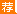# 2018陕西教师招聘考试信息技术学科-进制转换习题2018陕西教师招聘考试公告信息汇总|辅导课程 教师招聘交流QQ群| 微信号：sajsks

1.将十进制数12转换成二进制数，其值是( )。

A.1000 B.1010 C.1011 D.1100

1.【答案】D。解析：十进制转换为而进制采用的方法是除以2取余，倒序输出。即：

12÷2=6余0

6÷2=3余0

3÷2=1余1

1÷2=0余1

2.将二进制数10110101转换为十进制数是( )。

A.180 B.181 C.-180 D.-181

2.【答案】B。解析：二进制转换为十进制数的规则是“位权展开法”，即(10110101)2=1×27+0×26+1×25+1×24+0×23+1×22+0×21+1×20=181。

3.将八进制的7.416转换成二进制数为：( )。

A.111.10000111 B.101.10000110

C.111.00000101 C.101.10000111

3.【答案】A。解析：八进制数转换成二进制数的方法为“一位拆三位”，按此规则进行转换得到二进制数为111.10000111。

4.将16进制2AF转换成十进制数是( )。

A.786 B.689 C.382 D.687

4.【答案】D。解析：十六进制转换为十进制的方法是“按位权展开相加法”。本题的计算过程是2×162+10×161+15×160=687。

5.下面四个数中，数值最小的是( )。

A.(25)10 B.(30)8 C.(10110)2 D.(17)16

5.【答案】C。解析：采用位权展开法，(25)10=25，(30)8=3×81+0×80=24，(10110)2=1×24+1×22+1×21=22，(17)16=1×161+7×160=23。所以数值最小的是C选项。